# Microelectronics

## 03 MOSFET

### Prof. Dr. Jörg Vollrath

02 History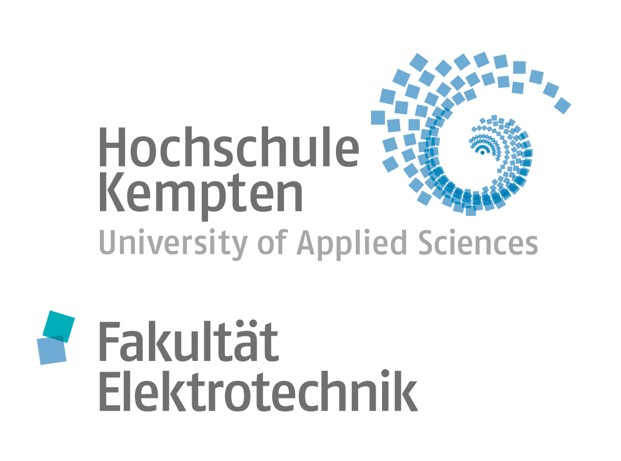## Video of lecture 03 MOSFET, SPICE models, propagation delay 31.03.2021

 Video is not visible, most likely your browser does not support HTML5 video Länge: 01:26:05 0:0:0 0 0:3:9 Goals today 0:6:36 Output and transfer curve 0:9:48 50 nm technology voltage limit 0:14:58 Subthreshold characteristic 0:16:49 Bulk source voltage and threshold voltage 0:19:25 Design by selecting W or number of unit transistors 0:24:29 MOSFET typical layout and rules 0:30:37 SPICE models 1 um, 50 nm 0:35:39 Infineon 130 nm MOSFET transistor parameters 0:40:29 SPICE models details 0:48:31 MOSFET as switch 0:50:39 Transistor RC model 0:52:39 Transistor Capacitances 0:59:19 Propagation delay 1:1:39 Rising and falling output 1:8:49 equivalent capacitance, Miller capacitance 1:16:49 Timing closure

# Overview

• Moores Law
• Microelectronic products, complexity, hierarchy
• Technologies: ASIC, FPGA, Microcontroller
• You have a basic understanding of a MOSFET
• Gate, Source, Drain, Bulk/Well: VGS, VDS, VBS
• Threshold voltage Vth, Drain source current IDS
• Transfer and output IV curve
• Output resistance RDSon
• Layout: Width, Length
• Capacitance and RDSon,C delay

# Microelectronic productsComputer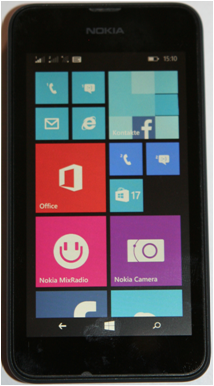mobile phone

# Hierarchical Description

 Top Down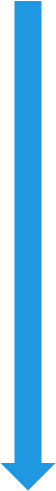System Subsystemi, Subsystemj Subsystemk, Subsysteml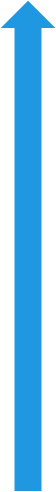Bottom up

# Citations

## Optimization

“The First Rule of Program Optimization: Don't do it.
The Second Rule of Program Optimization (for experts only!): Don't do it yet.”
- Michael A. Jackson

## Diagnose problems

“We should forget about small efficiencies, say about 97% of the time:
premature optimization is the root of all evil.
Yet we should not pass up our opportunities in that critical 3%.
A good programmer will not be lulled into complacency by such reasoning,
he will be wise to look carefully at the critical code;
but only after that code has been identified”
- Donald Knuth

# Design Flow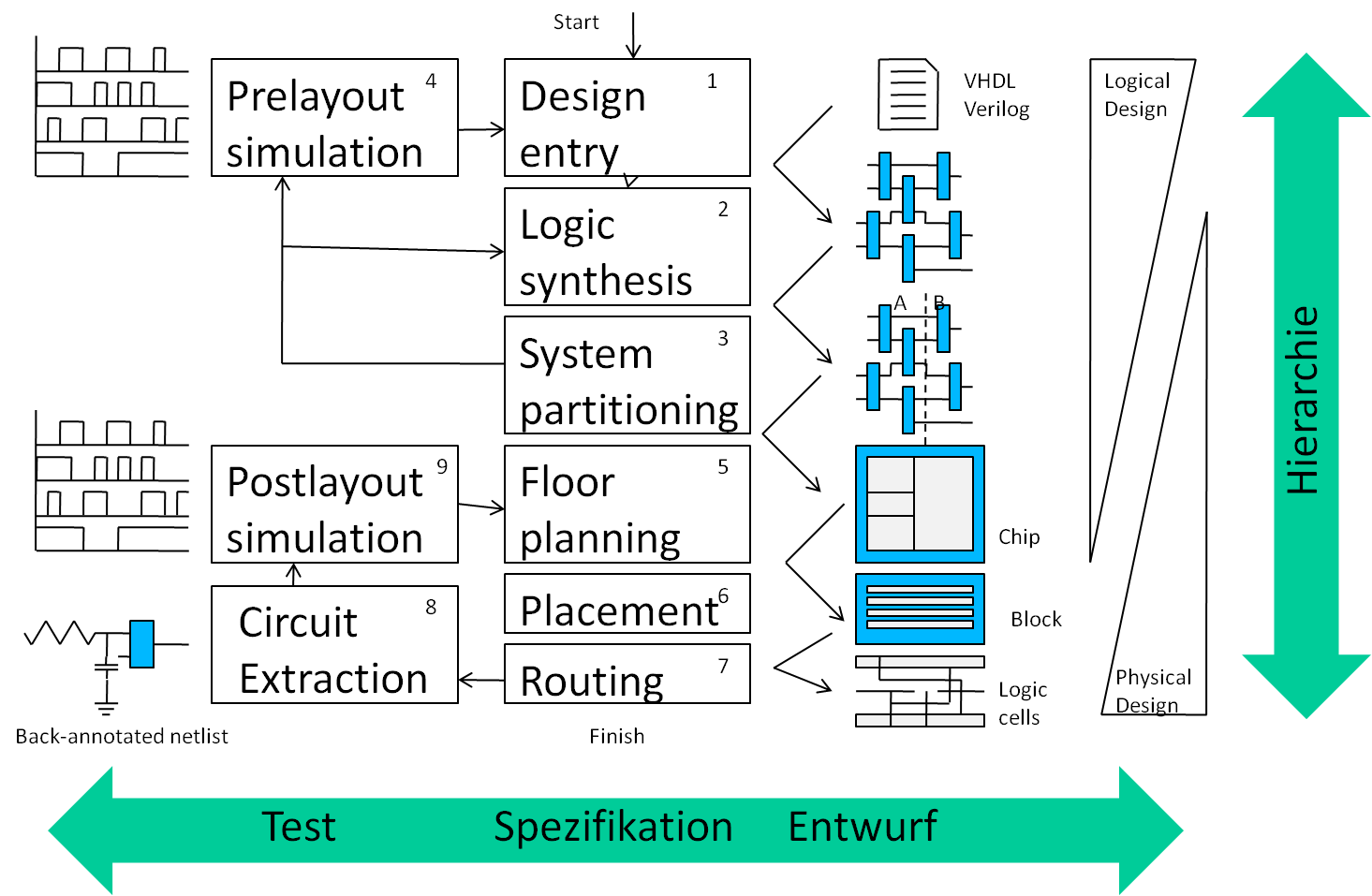# Technologies

Comparison## ASIC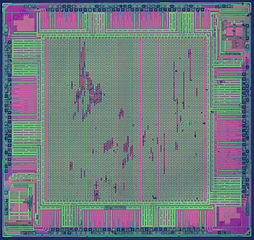## FPGA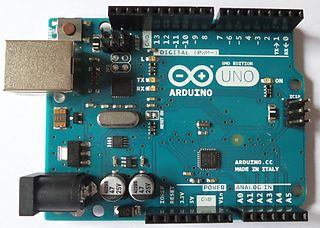## Microcontroller

Target market High volume Prototype to medium volume Single to high volume
Design speed Slow Fast Faster
Design Tools Expensive No cost No cost
Efficiency Optimum slow clock, parallel operation slow clock, seriell operation, analog function
ASIC: Application specific integrated circuit.
FPGA: File programmable gate array.

# Systems

## Raspberry Pi: µC, periphery

Operating system: Linux
Communication: Wifi, Webserver C(++), JavaScript
Application: C(++), JavaScript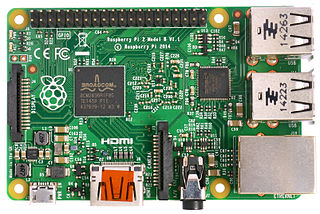## Arduino: Microcontroller (µC)

No operating system
Application and Communication C

## Zedboard: FPGA, µC

Field programmable gate array (FPGA)
FPGA programming: VHDL, Verilog, IP Wizard
Optional operating system: Linux
Communication: Wifi, Webserver C(++), JavaScript
Application: C(++), JavaScript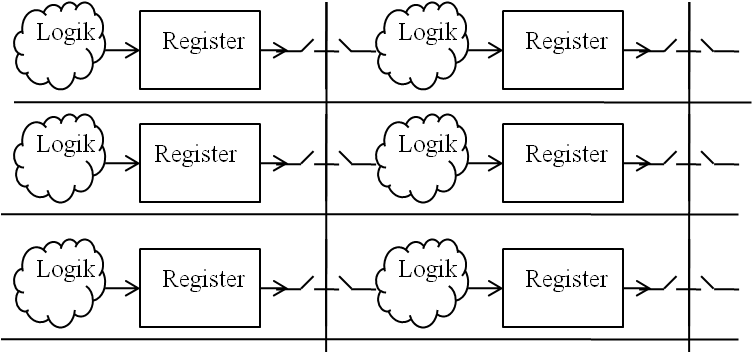Switch matrix, logic and registers, programmable

## ASIC

Proprietory software for design
Electronic design automation (EDA) software tools:
linux, perl, phyton, JavaScript
Full custom ASIC
Standard cell ASIC
Gate Array Based Asic

Foundries:
TSMC, UMC

Educational:
MOSIS
Europractice

Matlab, Labview can support some platforms.

# Electronic system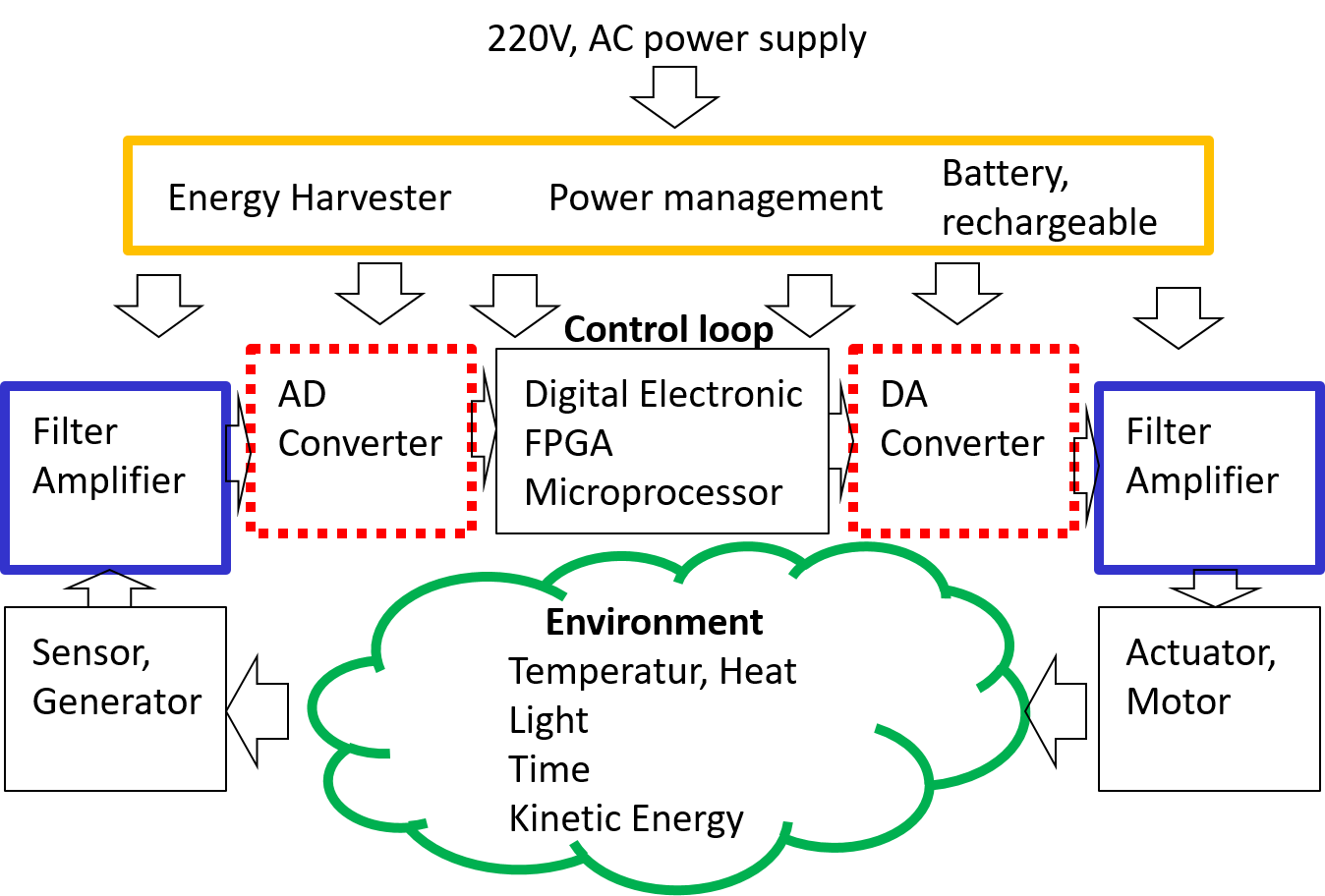# System Description

• State diagram, truth table
• Schematic (Graphic with symbols)
• Logic diagram
• Signal behaviour
• Hardware description language (HDL): Verilog, VHDL
• SystemC, Matlab, Simulink, Labview
• Programming Language: JavaScript

Modelling
Simulation

Structural Description
Behavioral Description

## Analog and digital signals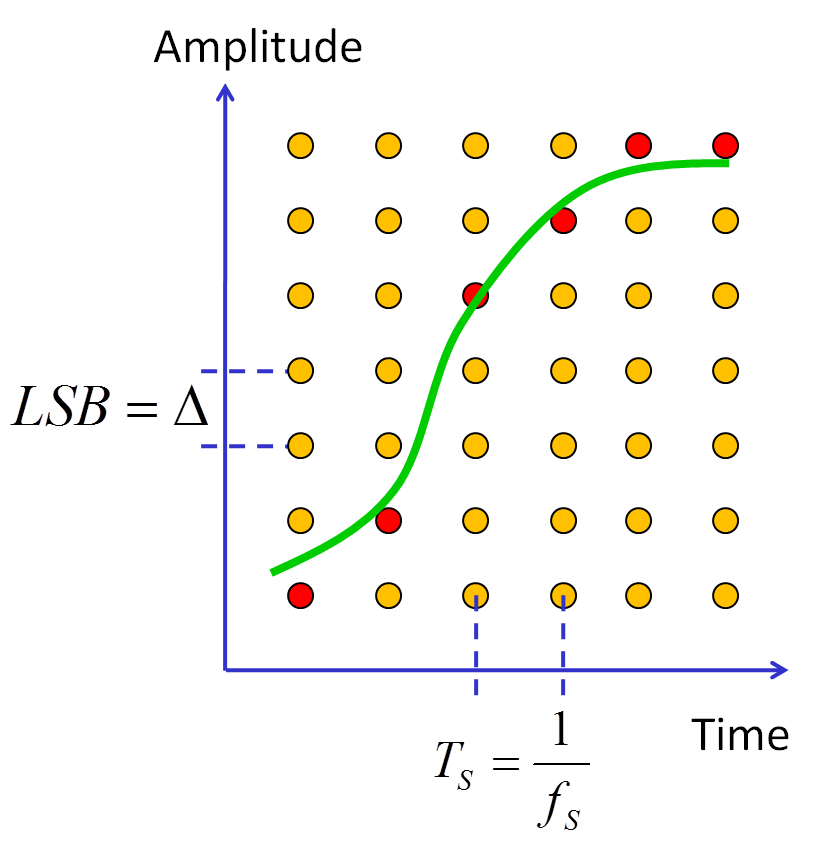# MOSFET Transistor

• Equations:
• Technology versus electrical properties
• Electrical properties for circuit simulation (SPICE program)
• 4 Terminal device
• Gate, source, drain, bulk (well)
• Voltage: VGS gate source voltage, VDS drain source voltage, VBS bulk source voltage
• Current: Gate, Drain source, bulk or substrate
• Electrical Characteristics
• Transfer Curve:
• lin IDS versus VGS -> shows threshold voltage
• log IDS versus VGS -> shows weak inversion and off current
• Output Curve: IDS versus VDS with various VGS -> shows output conductance
• Technology versus Electrical Characteristics: Roll of curve -> Vth versus Leff

# Transistor Evolution

 How much area uses one transistor? Theoretical minimum: Active area: W*L*4 Contacts: W*L*4*3 Minimum Feature size F A=16*F2 Example: F = 45 nm; 731M Transistors; 262 mm2 A = 262 mm2/(731*106)= 358413 nm2 = 177*F2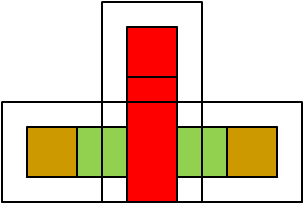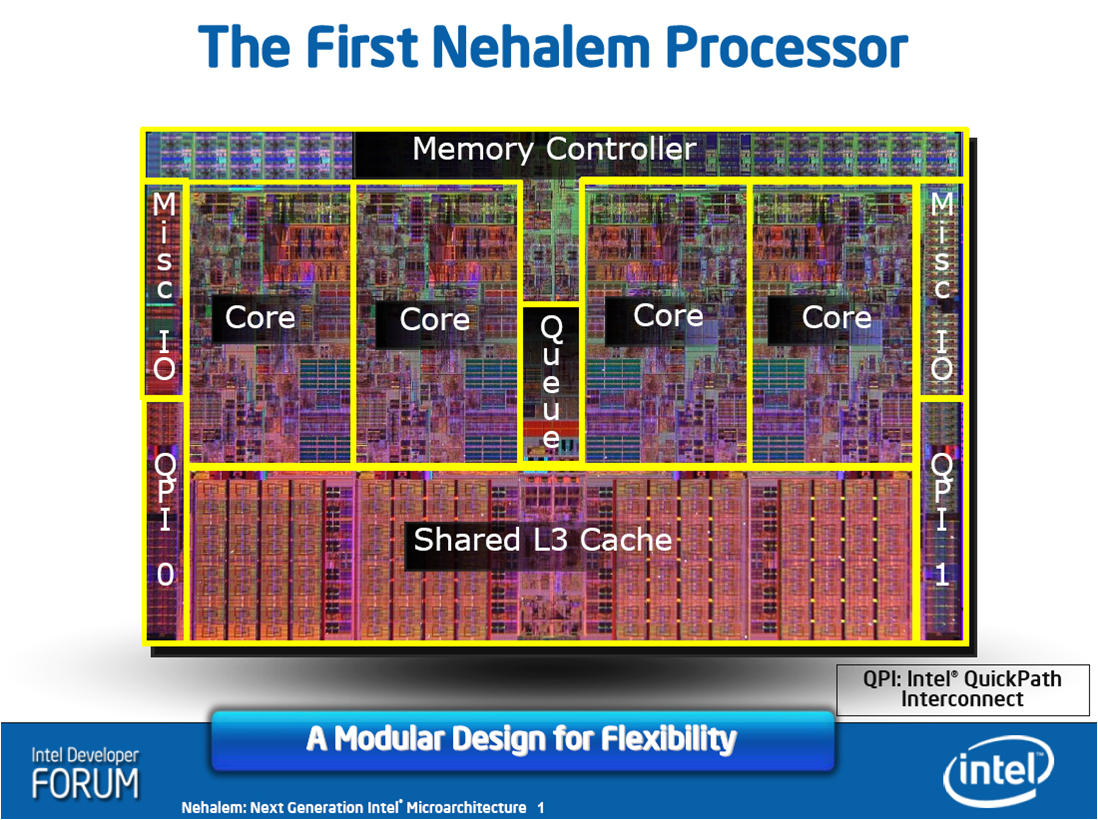Courtesy of Intel 2008 Intel Core i7 731 Million MOS Transistors 45 nm process 263 mm2 2.6..3.2 GHz

## Summary Transistor Evolution

MOSFET- transistor
Moore‘s law
Implications of reduced feature size:
30% Productivity gain per year
Power consumption, complexity/features, price
Hierarchical Design
Verification

Microelectronics deals with small electronic components
Transistor, diode, resistor, capacitor, inductance
Microelectronic systems are getting cheaper
Complex systems can be realized
Functions are getting cheaper

Typical microelectronic components of interest are:
Microcontroller (Microprocessor, memory, digital and analog functions)
FPGA (Field programmable gate arrays)
ASIC (Application specific integrated circuits)
This class shows how to design digital and analog CMOS microelectronic components and systems

# Inverter schematic and layout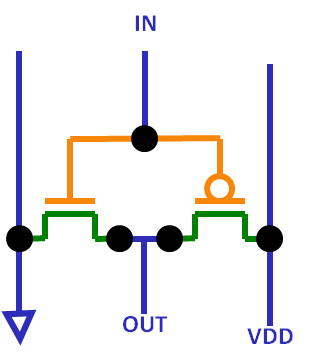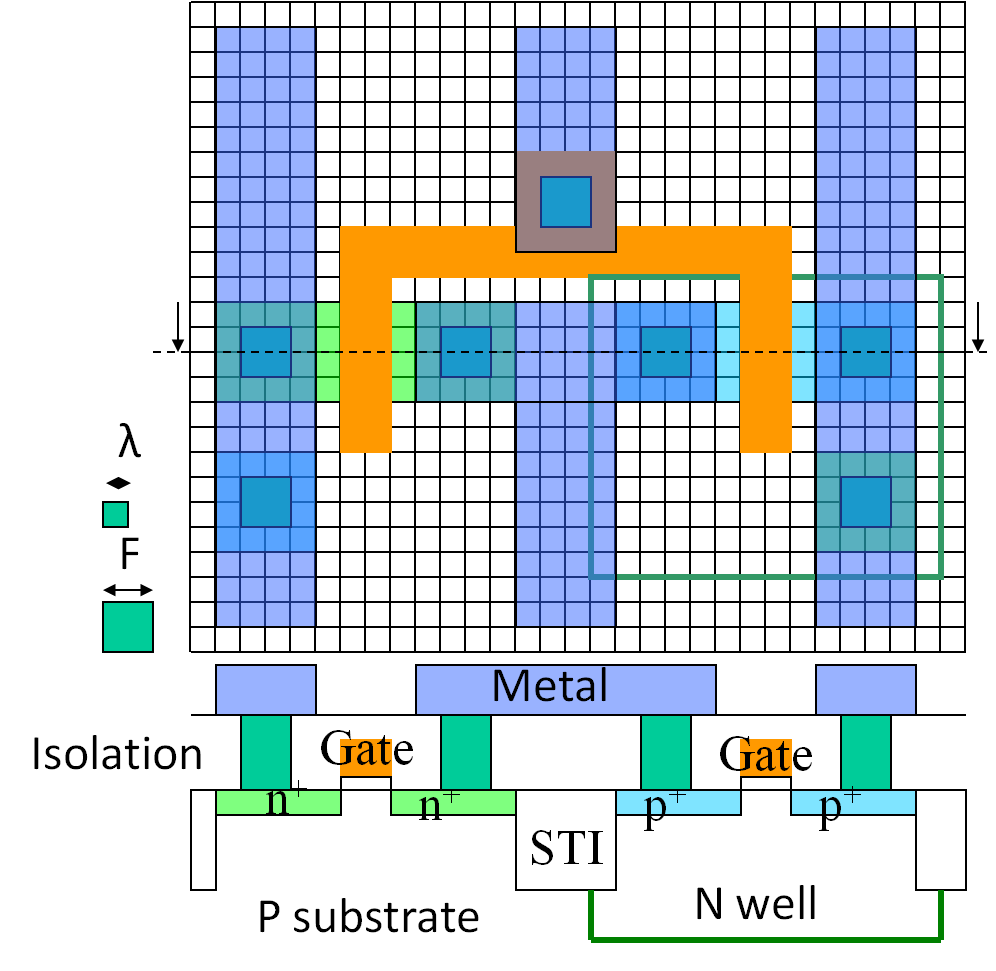Schematic and layout are representing an inverter.
An inverter uses a NMOSFET and a PMOSFET.
The schematic shows the components and connections.
The layout has added different colors for different layers of the manufacturing process and each component.
These colors are transferred into the schematic showing the relationship between layout and schematic.
Wiring and transistor gates and diffusion have a minimum physical size of width and length of F.
There are also minimum distances required to prevent shorts.

It is difficult to automatically transform one view into the other.
A schematic is drawn to be able to easily understand the circuit. On the left is GND, on the right VDD, on the top inputs, on the bottom outputs.
In a schematic connection between 2 pins can be accomplished by labeling the pins with the same names. Lines can cross without resulting in short circuits.
A layout is drawn for symmetric and regular patterns, avoiding signal cross coupling and shape distortion, and having minimum size.

# Equations of a n-channel MOSFET

 $I_{DS}= \cases{ \begin{matrix} 0 & \text{for} & V_{GS} \leq V_{th} & \text{Off} \\ I_{on} exp^{\frac{ V_{GS}-V_{th} - \frac{kT}{e}}{\frac{kT}{e}}} \left( 1+\lambda V_{DS} \right) & \text{for} & 0 \leq V_{GS} - V_{th} \lt \frac{kT}{e} & \text{Weak inversion} \\ \beta \left( V_{GS}-V_{th} \right)^2 \left( 1+\lambda V_{DS} \right) & \text{für} & \frac{kT}{e} \leq V_{GS} - V_{th} \lt V_{DS} & \text{Saturation} \\ \beta \left( 2 \left( V_{GS}-V_{th} \right) V_{DS} - V_{DS}^2 \right) & \text{for} & \frac{kT}{e} \leq V_{GS} - V_{th} \geq V_{DS} & \text{Linear} \end{matrix} }$ $\beta = \frac{\mu_n \epsilon_{ox}}{2d_{ox}} \frac{W}{L} = \frac{1}{2} \mu_n C_{ox}^{'} \frac{W}{L} = \frac{1}{2} K_{n}^{'} \frac{W}{L} = \frac{1}{2} K_{n}$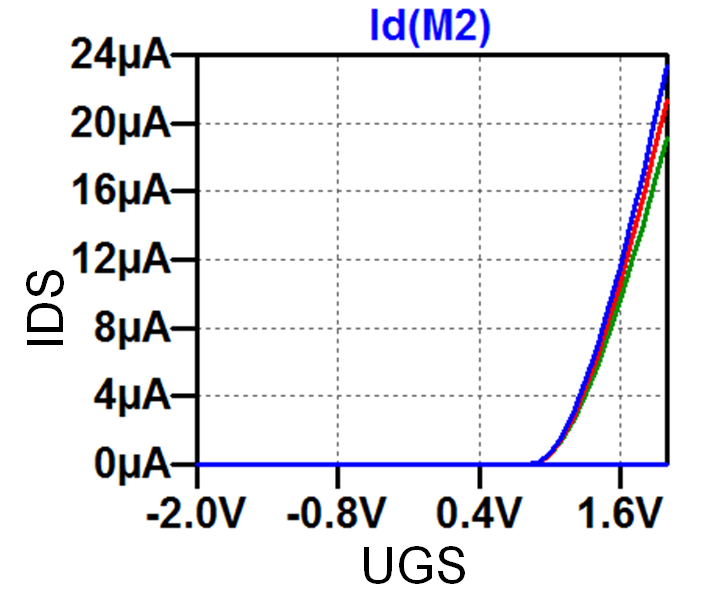Quelle Vollrath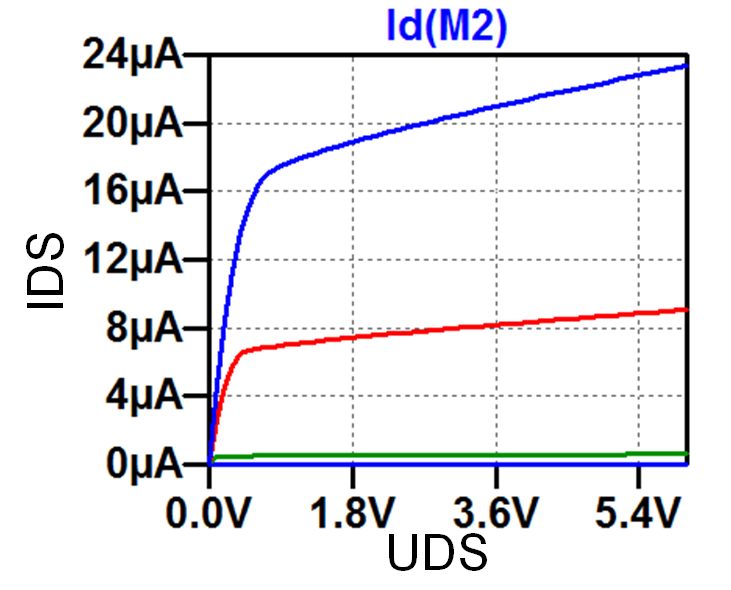Quelle Vollrath
Vth threshold voltage
λ Output conductance
W width, L length
µ mobility
Cox = e /dox Gate oxide capacitance
Vt = kT/q (300K) = 0.0259 V

Simulation verifies first correct Vth and maximum current IDS at maximum voltage Vdd.
Then the input capacitance Cox is confirmed with AC simulation of a RC circuit having the MOSFET gate as capacitance C.

Microelectronic has only transistors as elements.
Design is done using transistors with choosing W and L.
MOS transistors are also used as capacitances, resistors, diodes and lateral bipolar transistors.
In sub 50nm processes FINFETs are used having a fixed L and W. These transistors are called unit transistors. Circuits are designed combining transistors in series or parallel to get the desired W/L ratio. This makes automated design easier.

Elektronik 3, 08 MOSFET

# NFET Transistor subthreshold characteristic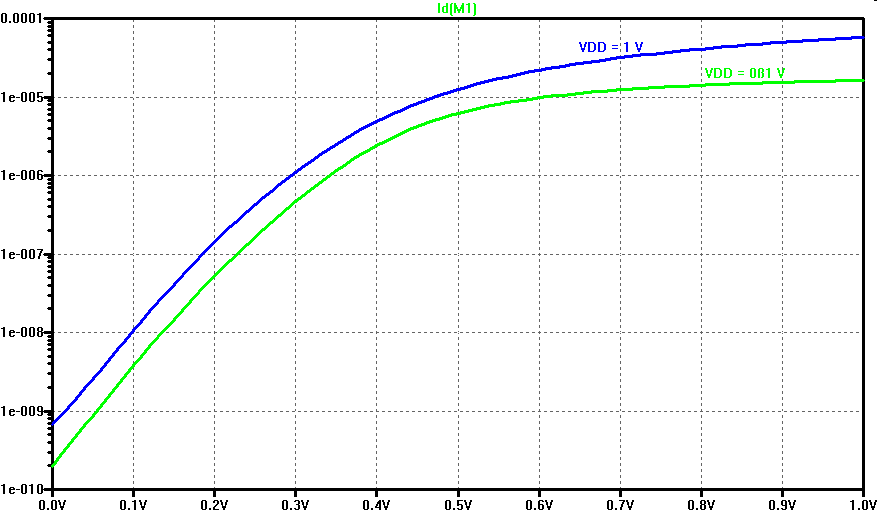MOSFET transistors can also be operated in the subthreshold region for low voltage power supply.
The graph shows an increase in current by a factor of 10 for 100 mV change in VGS.

# Threshold voltage Vth

 $V_{th}= V_{th0} + \left( \gamma \sqrt{V_{SB}+2\phi_F}-\sqrt{2\phi_F}\right)$
 Vth: Threshold voltage VSB: Source-bulk voltage $\phi_F$: Fermipotential of semiconductor $\gamma$: Body factor Graph: $V_{th0}=1V$ $\phi_{F}=0.3V$ $\gamma=0.75 \sqrt{V}$

# MOSFET Transistor design

• Choosing W and L for IDS
• L is fixed to minimum feature size
• W can be changed
• Unit transistors have a fixed W and L
Circuits are using unit transistors in parallel and series
• Threshold voltage Vth
• Vth is fixed for a given process
• Vth can change with:
oxide thickness, doping, length and width
• Modern processes have different devices:
High Vth, low Vth, thick and thin oxide devices
• Process sensitivity analysis: Process window lot (PWL)
Check in simulation different Vth and W L variations
• # MOSFET layout

 Simple: crossing of polysilicon and contact diffusion Finger structure Serpentine Common centroid layout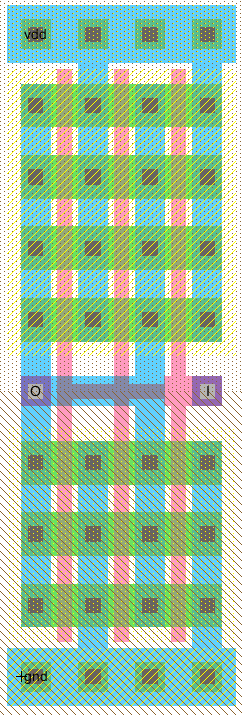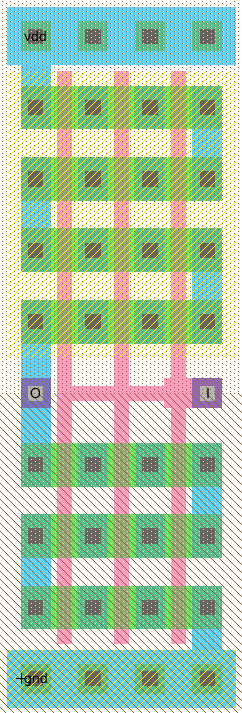# MOSFET SPICE models

 Long channel MOSFET F = 1 µm, VDD = 5 V Parameter NMOS PMOS Comment VTh 0.8 V 0.9 V Typical KP 120 µA/V2 40 µA/V2 dox = 20 nm C'ox 1.75 fF/µm2 1.75 fF/µm2 Cox = C'ox W L λ 0.01 V-1 0.0125 V-1 L = 2 R 15 kΩ L/W 45 kΩ L/W Short channel MOSFET F = 50 nm, VDD = 1 V Parameter NMOS PMOS Comment VTh 0.28 V 0.28 V Typical KP 60 µA/V2 30 µA/V2 dox = 1.4 nm C'ox 25 fF/µm2 25 fF/µm2 Cox = C'ox WL λ 0.6 V-1 0.3 V-1 L = 2 R 34 kΩ/W 68 kΩ/W
Table according to Baker. Width W and L length are applied in multiples of feature size F for KP.

Propagation delay can be calculated with tPD = 0.7 · R · C
R is the resistance of NFET or PFET. C is the sum of the output capacitance (Coxn) and input capacitance (3/2 Cox).
C = (3/2 + 1 ) (Coxn + Coxp)

A 1 µm unit transistor has a Cox of 1.75 fF and R of 15 kΩ (45 kΩ).
tPD = 0.7 · 2 · 1.75 fF · 0.5 · ( 15 kΩ + 45 kΩ) = 73.5 ps

A 50 nm unit transistor has a Cox of 25 fF/µm2 · 50nm · 50 nm = 62.5 aF and R of 34 kΩ (68 kΩ).
tPD = 0.7 · 2 · 25 fF · 50 nm · 50 nm · 0.5 · ( 34 kΩ + 68 kΩ) = 2.23 ps

Simulated inverter 24..32 ps

More models:

Baker: 1 µm MOSFET model, 50 nm MOSFET model, cmosedu_models.txt
Sedra Smith Level=1 5 µm, 0.5 µm: sedra_lib.lib
Allen, Holberg Level=3 0.8 µm: Holberg.txt
TSMC 0.25um CMOS MOSFETs (level 3) t14y_tsmc_025_level3.lib from MOSIS .

#### CD4007

.model CD4007N NMOS(LEVEL=1 KP=500u VT0=1 LAMBDA=0.002 CGSO=45n CGBO=2n CGDO=45n)
.model CD4007P PMOS(LEVEL=1 KP=500u VT0=-1 LAMBDA=0.002 CGSO=45n CGBO=2n CGDO=45n)

#### ALD1106, ALD1107

.model ALD1106N NMOS(LEVEL=1 KP=0.48m VT0=0.7 LAMBDA=0.018 CGDO=100n CGSO=100n)
.model ALD1107P PMOS(LEVEL=1 KP=0.2m VT0=-0.7 LAMBDA=0.018 CGDO=100n CGSO=100n)

# 130 nm Infineon MOSFET transistors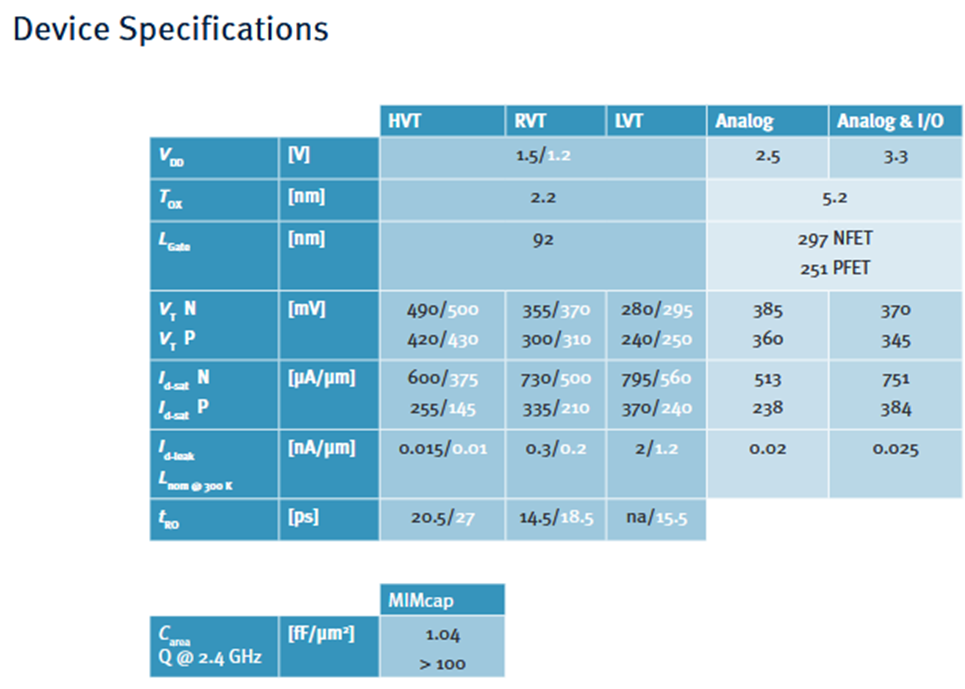Infineon 130 nm platform

04 Inverter